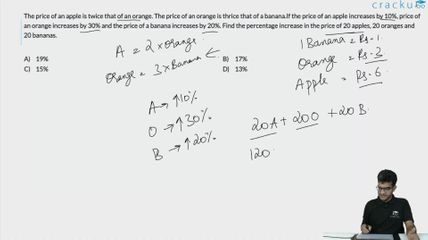Question 7

# The price of an apple is twice that of an orange. The price of an orange is thrice that of a banana.If the price of an apple increases by 10%, price of an orange increases by 30% and the price of a banana increases by 20%. Find the percentage increase in the price of 20 apples, 20 oranges and 20 bananas.

Solution

Let price of banana be x
so price of orange = 3x
and price of apple will be 6x
Price of 20 bananas , apples and oranges = 200 x  (1)
Now f the price of an apple increases by 10%,
it becomes = 6.6x
so price of 20 apples = 132x
if price of an orange increases by 30% it becomes = 3.9x
so price of 20 oranges = 78x
if price of a banana increases by 20%
it becomes 1.2 x
so price of 20 bananas = 24x
Now total price of 20 bananas , apples and oranges =234x  (2)
So Percentage change in price = $$\frac{234x-200x}{200x}\times\ 100=\ 17$$%

### View Video Solution